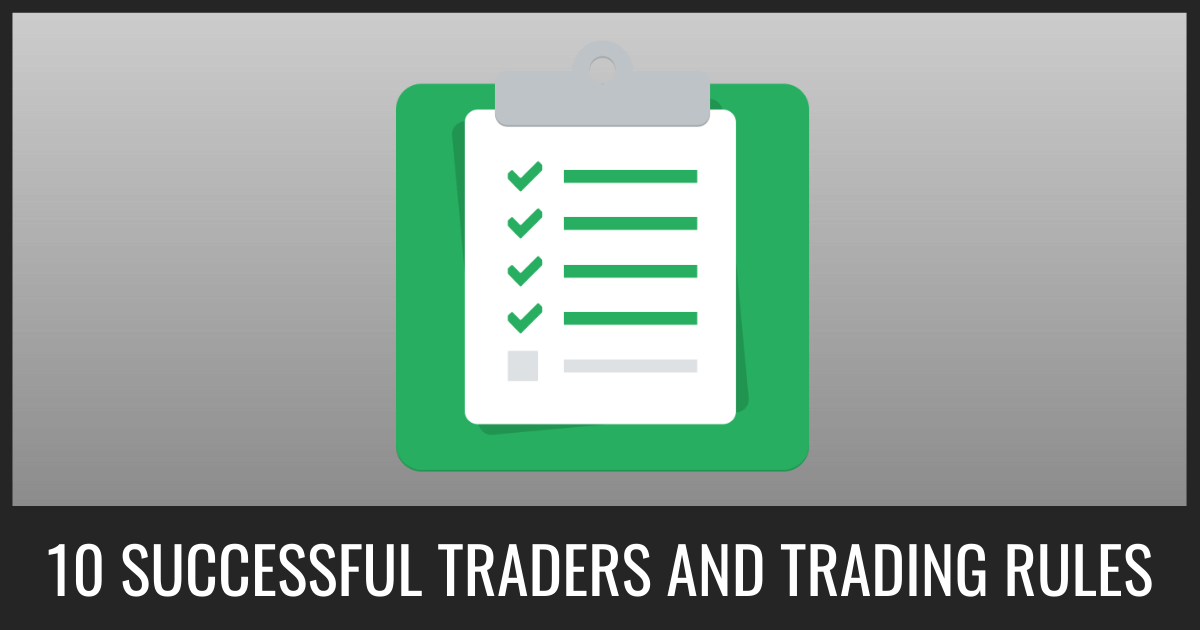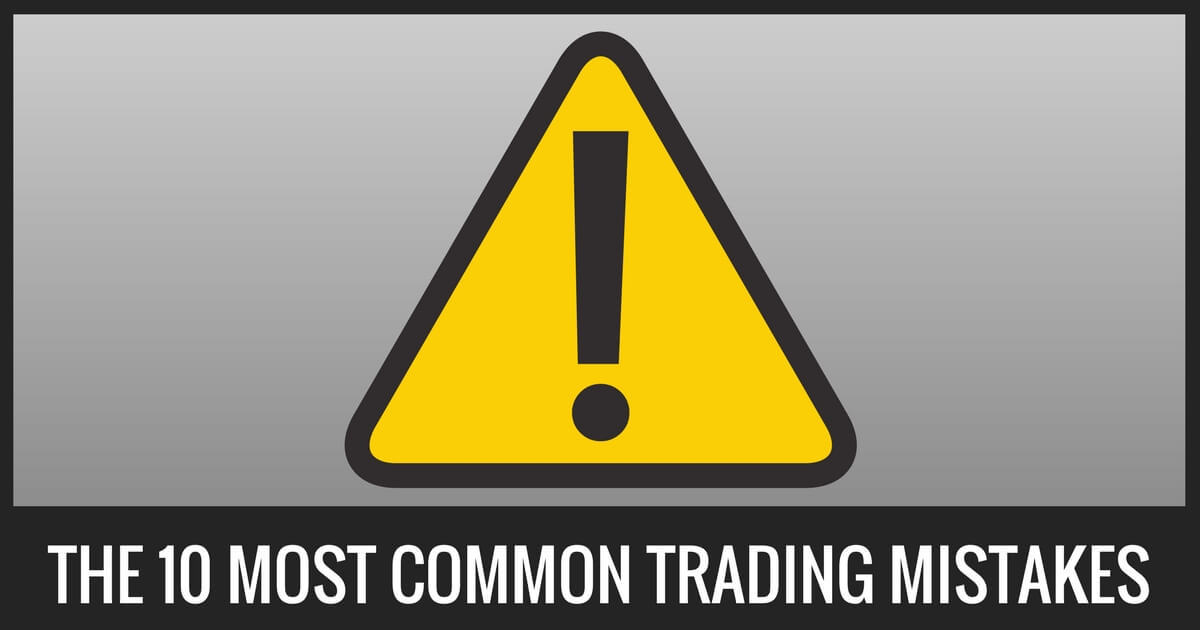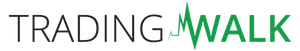# The Best Fibonacci Retracement Trading Strategy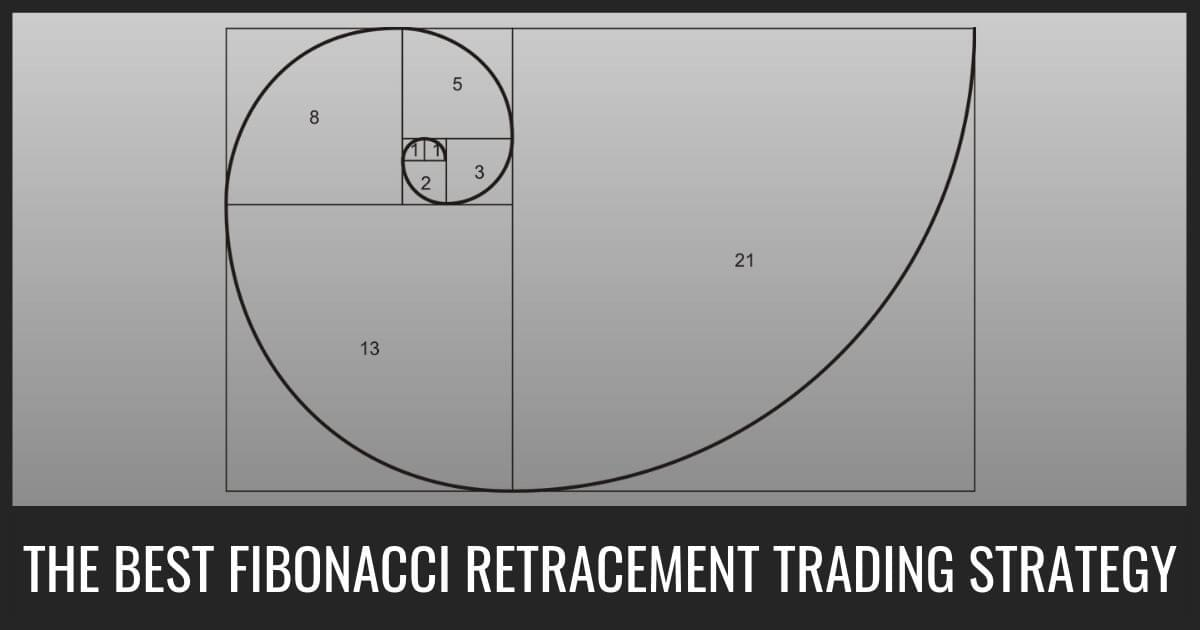Ready to learn how to trade Fibonacci retracement levels in an easy and profitable way?

In mathematics, the Fibonacci numbers are the numbers in the following integer sequence, called the Fibonacci sequence, and characterized by the fact that every number after the first two is the sum of the two preceding ones.

In technical analysis, Fibonacci retracement levels are created by taking two extreme points (usually a major swing high and swing low) on a chart and dividing the vertical distance by the key Fibonacci ratios of 23.6%, 38.2%, 50%, 61.8% and 100%.

# Why Fibonacci Retracement Works

The major reason why Fibonacci retracement works is because the patterns appear in nature and many traders swear by these support and resistance levels.

When a Fibonacci retracement level is identified on a higher timeframe chart, you can be sure that a large number of traders will be watching that level, many of them having existing orders around the strong level.

The Fibonacci retracement levels are some of the best support and resistance trading levels.

How and where to enter trades using Fibonacci retracement levels gets really easy when you’re using the simple Fibonacci trading strategy in the video above.

The most important Fibonacci ratios/retracements are the 38,2% the 50% and the 61,8% levels.

Remember; trading strategies you trade in the direction of the trend will give you a good risk-reward and high win rate.

A good strategy to trade the retracement levels is to wait for a candlestick patten to confirm the level before you take a position.

# Fibonacci Forex Strategy Examples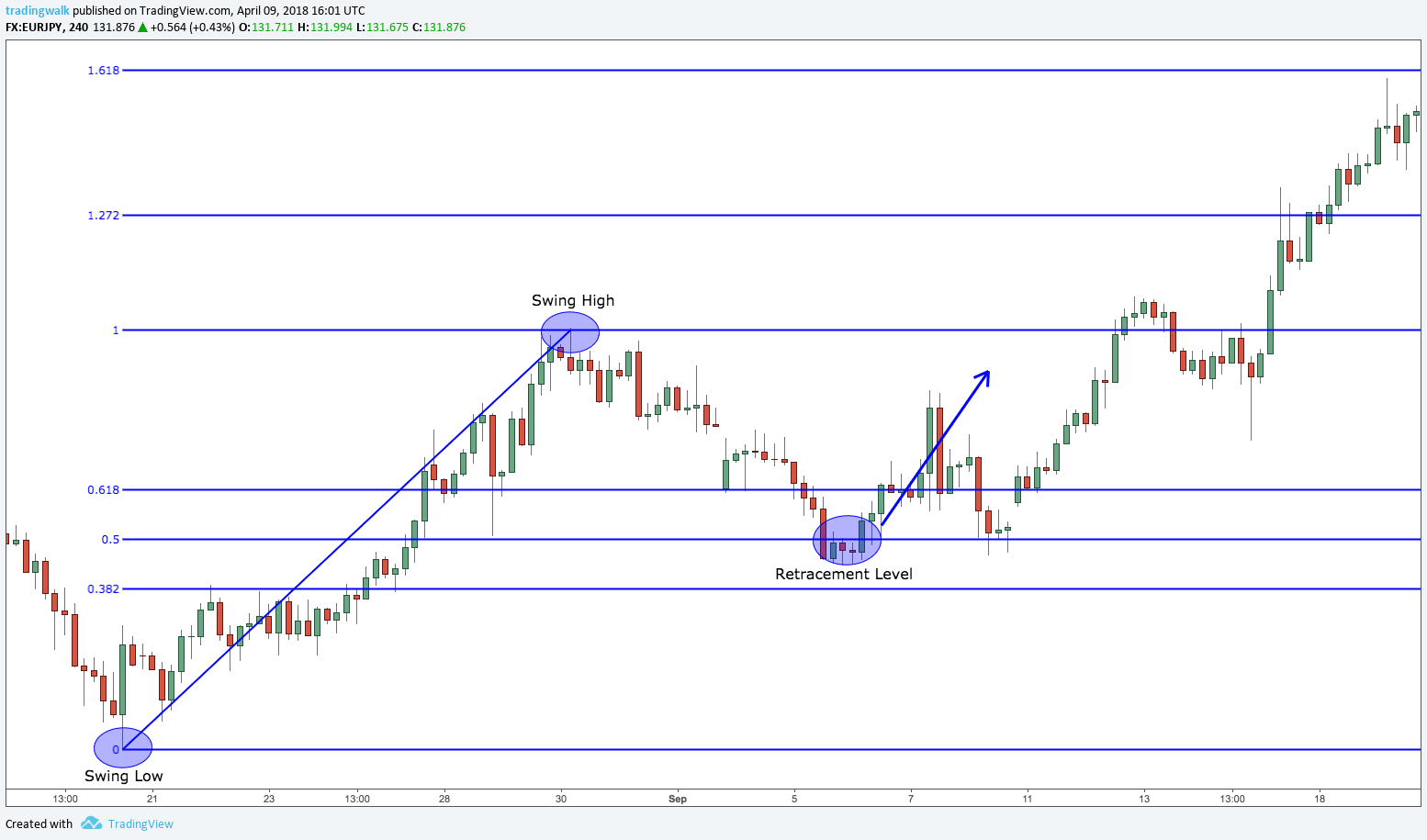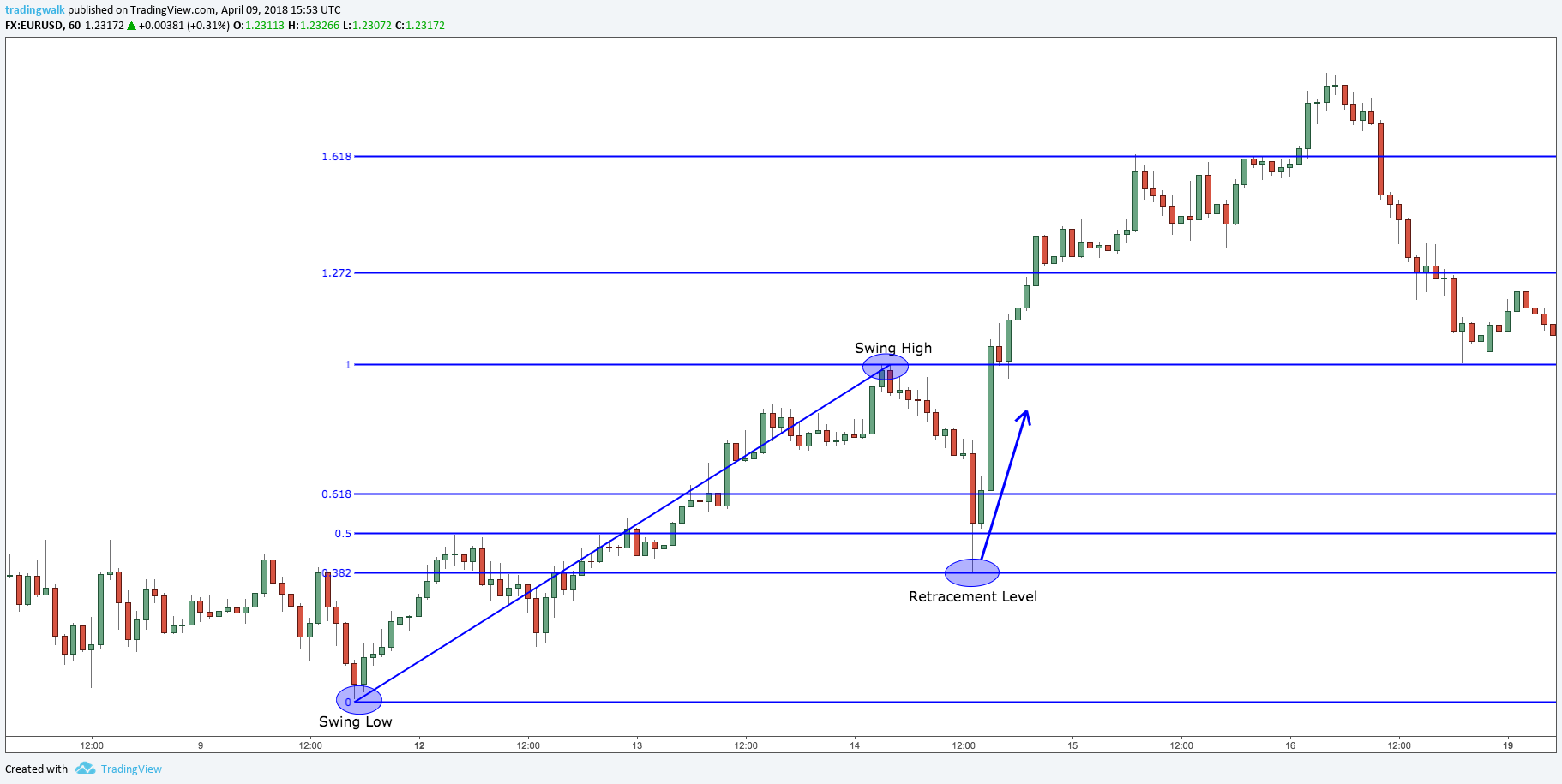CUSTOM JAVASCRIPT / HTML
• ​Find recent swing highs and swing lows on your chart.
• ​Draw the Fibonacci indicator between recent high and low.
• ​Trade in the direction of the trend.
• ​When resistance levels break (uptrend, new highs are formed), you want to enter on the first test of a Fibonacci retracement support level that you have drawn between the most recent high and low.
• ​When support levels break (downtrend, new lows are formed), you want to enter on the first test of Fibonacci retracement resistance level that you have drawn between the most recent high and low.
CUSTOM JAVASCRIPT / HTML
Do You Want My
FREE Forex Strategy?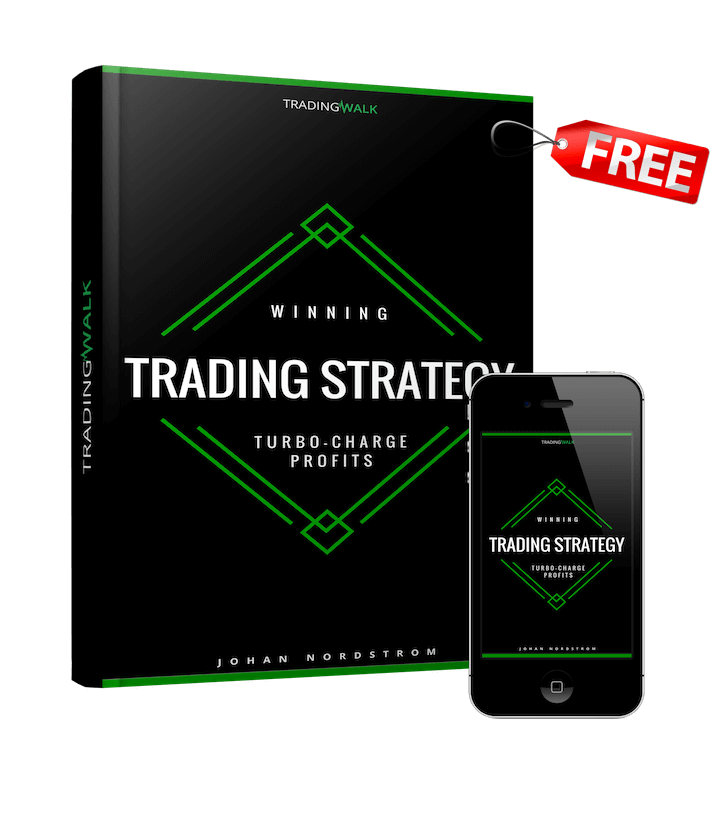I'm a family guy in my late 20's who learned how to trade the markets in a simple yet effective way. During university I studied investing and graduated with a master's degree in risk management. Quickly, I realized that I was onto something. I started helping friends and taking students. My students started getting results, spent less time in front of their screens, and their accounts grew consistently. Learn more about me here.
The Most Important
FX Tools and Resources
You Might Also Like...